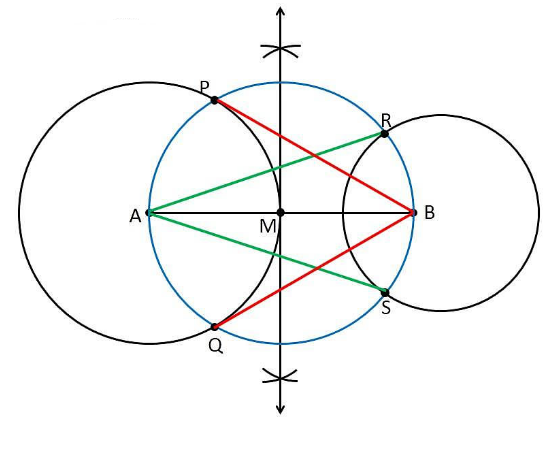Guru

# Draw a line segment AB of length 8 cm. Taking A as centre, draw a circle of radius 4 cm and taking B as centre, draw another circle of radius 3 cm. Construct tangents to each circle from the centre of the other circle. Q.5

• 0

How i solve the problem of class 10th ncert math of exercise 11.2 of question no,5 of constructions chapter, give me the best and the easy way to solve this problem Draw a line segment AB of length 8 cm. Taking A as centre, draw a circle of radius 4 cm and taking B as centre, draw another circle of radius 3 cm. Construct tangents to each circle from the centre of the other circle.

Share

1. Construction Procedure:

The tangent for the given circle can be constructed as follows.

1. Draw a line segment AB = 8 cm.

2. Take A as centre and draw a circle of radius 4 cm

3. Take B as centre, draw a circle of radius 3 cm

4. Draw the perpendicular bisector of the line AB and the midpoint is taken as M.

5. Now, take M as centre draw a circle with the radius of MA or MB which the intersects the circle at the points P, Q, R and S.

6. Now join AR, AS, BP and BQ

7. Therefore, the required tangents are AR, AS, BP and BQJustification:

The construction can be justified by proving that AS and AR are the tangents of the circle (whose centre is B with radius is 3 cm) and BP and BQ are the tangents of the circle (whose centre is A and radius is 4 cm).

From the construction, to prove this, join AP, AQ, BS, and BR.

∠ASB is an angle in the semi-circle. We know that an angle in a semi-circle is a right angle.

∴ ∠ASB = 90°

⇒ BS ⊥ AS

Since BS is the radius of the circle, AS must be a tangent of the circle.

Similarly, AR, BP, and BQ are the required tangents of the given circle.

• 0